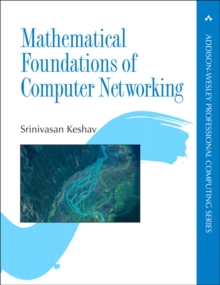Supporting your high street Find out how »
• My AccountMathematical Foundations of Computer Networking Paperback / softback

Description

"To design future networks that are worthy of society's trust, we must put the `discipline' of computer networking on a much stronger foundation.

This book rises above the considerable minutiae of today's networking technologies to emphasize the long-standing mathematical underpinnings of the field."-Professor Jennifer Rexford, Department of Computer Science, Princeton University "This book is exactly the one I have been waiting for the last couple of years.

Recently, I decided most students were already very familiar with the way the net works but were not being taught the fundamentals-the math.

This book contains the knowledge for people who will create and understand future communications systems."-Professor Jon Crowcroft, The Computer Laboratory, University of Cambridge The Essential Mathematical Principles Required to Design, Implement, or Evaluate Advanced Computer Networks Students, researchers, and professionals in computer networking require a firm conceptual understanding of its foundations.

Mathematical Foundations of Computer Networking provides an intuitive yet rigorous introduction to these essential mathematical principles and techniques. Assuming a basic grasp of calculus, this book offers sufficient detail to serve as the only reference many readers will need.

Each concept is described in four ways: intuitively; using appropriate mathematical notation; with a numerical example carefully chosen for its relevance to networking; and with a numerical exercise for the reader. The first part of the text presents basic concepts, and the second part introduces four theories in a progression that has been designed to gradually deepen readers' understanding.

Within each part, chapters are as self-contained as possible. The first part covers probability; statistics; linear algebra; optimization; and signals, systems, and transforms.

Topics range from Bayesian networks to hypothesis testing, and eigenvalue computation to Fourier transforms. These preliminary chapters establish a basis for the four theories covered in the second part of the book: queueing theory, game theory, control theory, and information theory.

The second part also demonstrates how mathematical concepts can be applied to issues such as contention for limited resources, and the optimization of network responsiveness, stability, and throughput.

Information

£62.99

£52.15

on all orders

Pick up orders

from local bookshops